Share

# RS Aggarwal solutions for Secondary School Class 10 Maths chapter 17 - Perimeter and Areas of Plane Figures [Latest edition]

Course
Textbook page

#### Chapters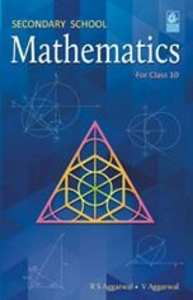## Chapter 17: Perimeter and Areas of Plane Figures

#### RS Aggarwal solutions for Secondary School Class 10 Maths Chapter 17 Perimeter and Areas of Plane Figures

Q 1

Find the area of triangle whose base measures 24 cm and the corresponding height measure 14.5 cm.

Q 2

Find the areas of the triangle whose sides are 42 cm, 34 cm and 20 cm. Also, find the height corresponding to the longest side.

Q 3

Find the area of the triangle whose sides are 18 cm, 24 cm and 30 cm. Also find the height corresponding to the smallest side.

Q 4

The sides of a triangle are in the ratio 5:12:13 and its perimeter is 150 m. Find the area of the triangle.

Q 5

The perimeter of a triangular field is 240m, and its sides are in the ratio 25:17:12. Find the area of the field. Also, find the cost of ploughing the field at ₹ 40 per m^2

Q 6

The perimeter of a right triangle is 40 cm and its hypotenuse measure 17 cm. Find the area of the triangle.

Q 7

The difference between the sides at the right angles in a right-angled triangle is 7 cm. the area of the triangle is 60 cm^2 . Find its perimeter.

Q 8

The length of the two sides of a right triangle containing the right angle differ by 2 cm. If the area of the triangle is 24xm^2, find the perimeter of the triangle.

Q 9

Each side of an equilateral triangle is 10 cm. Find (i) the area of the triangle and (ii) the height of the triangle.

Q 10

The height of an equilateral triangle is 6 cm. Find its area.

Q 11

If the area of an equilateral triangle is  36sqrt3 cm^2 find its perimeter.

Q 12

If the area of an equilateral triangle is 81sqrt3 cm^2 find its height.

Q 13

The base of a right – angled triangle measures 48 cm and its hypotenuse measures 50 cm. Find the area of the triangle.

Q 14

The hypotenuse of a right-angled triangle is 65 cm and its base is 60 cm. Find the length of perpendicular and the area of the triangle.

Q 15

Find the area of a right – angled triangle, the radius of whose, circumference measures 8 cm and the altitude drawn to the hypotenuse measures 6 cm.

Q 16

Find the length of the hypotenuse of an isosceles right-angled triangle whose area is 200^2 cm . Also, find its perimeter

Q 17

The base of an isosceles triangle measures 80 cm and its area is 2 360 . cm^2 Find the perimeter of the triangle.

Q 18

Each of the equal sides of an isosceles triangle measure 2 cm more than its height, and the base of the triangle measure 12 cm. Find the area of the triangle.

Q 19

Find the area and perimeter of an isosceles right angled triangle, each of whose equal sides measure 10cm.

Q 20

In the given figure, ΔABC  is an equilateral triangle the length of whose side is equal to 10 cm, and ΔADC is right-angled at D and BD= 8cm. Find the area of the shaded region.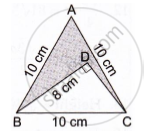#### RS Aggarwal solutions for Secondary School Class 10 Maths Chapter 17 Perimeter and Areas of Plane Figures

Q 1

The perimeter of a rectangular plot of land is 80 m and its breadth is 16 m. Find the length and area of the plot.

Q 2

The length of a rectangular park is twice its breadth and its perimeter is 840 m. Find the area of the park.

Q 3

One side of a rectangle is 12 cm long and its diagonal measure 37 cm. Find the other side and the area of the rectangle.

Q 4

The area of a rectangular plot is  462m^2 and is length is 28 m. Find its perimeter.

Q 5

A lawn is in the form of a rectangle whose sides are in the ratio 5 : 3. The area of the lawn is 3375m^2 . Find the cost of fencing the lawn at ₹ 65 per metre.

Q 6

A room is 16 m long and 13.5 m broad. Find the cost of covering its floor with 75-m-wide carpet at ₹ 60 per metre.

Q 7

The floor of a rectangular hall is 24 m long and 18 m wide. How many carpets, each of length 2.5 m and breadth 80 cm, will be required to cover the floor of the hall?

Q 8

A 36-m-long, 15-m-borad verandah is to be paved with stones, each measuring 6dm by 5 dm. How many stones will be required?

Q 9

The area of rectangle is 192cm^2 and its perimeter is 56 cm. Find the dimensions of the rectangle.

Q 10

A rectangular park 358 m long and 18 m wide is to be covered with grass, leaving 2.5 m uncovered all around it. Find the area to be laid with grass.

Q 11

A rectangular plot measure 125 m by 78 m. It has gravel path 3 m wide all around on the outside. Find the area of the path and the cost of gravelling it at ₹ 75    per   m^2

Q 12

A footpath of uniform width runs all around the inside of a rectangular field 54m long and 35 m wide. If the area of the path is   420m^2 , find the width of the path.

Q 13

The length and breadth of a rectangular garden are in the ratio 9:5. A path 3.5 m wide, running all around inside it has an area of  1911m^2 . Find the dimensions of the garden.

Q 14

A room 4.9 m long and 3.5 m board is covered with carpet, leaving an uncovered margin of 25 cm all around the room. If the breadth of the carpet is 80 cm, find its cost at ₹ 80 per metre.

Q 15

A carpet is laid on floor of a room 8 m by 5 m. There is border of constant width all around the carpet. If the area of the border is 12m^2 find its width.

Q 16

A 80 m by 64 m rectangular lawn has two roads, each 5 m wide, running through its middle, one parallel to its length and the other parallel to its breadth. Find the cost of gravelling the reads at ₹   40 per    m^2

Q 17

The dimensions of a room are 14 m x 10 m x 6.5 m There are two doors and 4 windows in the room. Each door measures 2.5 m x 1.2 m and each window measures 1.5 m x 1 m. Find the cost of painting the four walls of the room at ₹  35perm^2 .

Q 18

The cost of painting the four walls of a room 12 m long at ₹ 30   per   m^2 is  ₹ 7560   per    m^2 and the cost of covering the floor with the mat at ₹  25   per   m^2 is   ₹ 2700 . find the dimensions of the room.

Q 19

Find the area and perimeter of a square plot of land whose diagonal is 24 m long.

Q 20

Find the length of the diagonal of a square whose area is 128 cm^2 Also, find its perimeter.

Q 21

The area of a square filed is 8 hectares. How long would a man take to cross it diagonally by walking at the rate of 4 km per hour?

Q 22

The cost of harvesting a square field at ₹ 900 per hectare is ₹ 8100. Find the cost of putting a fence around it at ₹ 18 per meter.

Q 23

The cost of fencing a square lawn at ₹ 14 per meter is ₹ 28000. Find the cost of mowing the lawn at ₹ 54  100 per m^2

Q 24

In the given figure ABCD is quadrilateral in which diagonal BD = 24 cm, AL ⊥ BD  and CM ⊥ BD  such that AL = 9cm and CM = 12 cm. Calculate the area of the quadrilateral.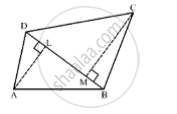Q 25

Find the area of the quadrilateral ABCD in which AD = 24 cm, ∠BAD 90° and ∠BCD  is an equilateral triangle having each side equal to 26 cm. Also, find the perimeter of the quadrilateral.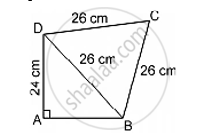Q 26

Find the perimeter and area of the quadrilateral ABCD in which AB = 17 cm, AD = 9 cm, CD = 12 cm, ∠ACB=90° and AC = 15 cm.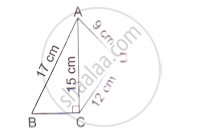Q 27

Find the area of the quadrilateral ABCD in which in AB=42 cm, BC=21 cm, CD=29cm, DA=34 cm and diagonal BD = 20 cm.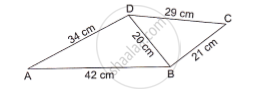Q 28

Find the area of a parallelogram with base equal to 25 cm and the corresponding height measuring 16.8 cm.

Q 29

The adjacent sides of a parallelogram are 32 cm and 24 cm. If the distance between the longer sides is 17.4 cm, find the distance between the shorter sides.

Q 30

The area of a parallelogram is 392m^2 . If its altitude is twice the corresponding base, determined the base and the altitude.

Q 31

The adjacent sides of a parallelogram ABCD measure 34 cm and 20 cm, and the diagonal AC measures 42 cm. Find the area of the parallelogram.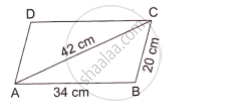Q 32

Find the area of the rhombus, the length of whose diagonals are 30 cm and 16 cm. Also, find the perimeter of the rhombus.

Q 33

The perimeter of a rhombus is 60 cm. If one of its diagonal us 18 cm long, find

(i) the length of the other diagonal, and

(ii) the area of the rhombus.

Q 34

The area of rhombus is  480cm^2 , and one of its diagonal measures 48 cm. Find

(i) the length of the other diagonal,

(ii) the length of each of the sides

(iii) its perimeter

Q 35

The parallel sides of trapezium are 12 cm and 9cm and the distance between them is 8 cm. Find the area of the trapezium.

Q 36

The shape of the cross section of a canal is a trapezium. If the canal is 10 m wide at the top, 6 m wide at the bottom and the area of its cross section is  640m^2 , find the depth of the canal.

Q 37

Find the area of trapezium whose parallel sides are 11 m and 25 m long, and the nonparallel sides are 15 m and 13 m long.

## Chapter 17: Perimeter and Areas of Plane Figures## RS Aggarwal solutions for Secondary School Class 10 Maths chapter 17 - Perimeter and Areas of Plane Figures

RS Aggarwal solutions for Secondary School Class 10 Maths chapter 17 (Perimeter and Areas of Plane Figures) include all questions with solution and detail explanation. This will clear students doubts about any question and improve application skills while preparing for board exams. The detailed, step-by-step solutions will help you understand the concepts better and clear your confusions, if any. Shaalaa.com has the CBSE Secondary School Class 10 Maths solutions in a manner that help students grasp basic concepts better and faster.

Further, we at Shaalaa.com provide such solutions so that students can prepare for written exams. RS Aggarwal textbook solutions can be a core help for self-study and acts as a perfect self-help guidance for students.

Concepts covered in Secondary School Class 10 Maths chapter 17 Perimeter and Areas of Plane Figures are Areas of Sector and Segment of a Circle, Areas Related to Circles Examples and Solutions, Perimeter and Area of a Circle, Areas of Combinations of Plane Figures, Problems Based on Areas and Perimeter Or Circumference of Circle, Sector and Segment of a Circle, Areas of Sector and Segment of a Circle, Areas Related to Circles Examples and Solutions, Perimeter and Area of a Circle, Areas of Combinations of Plane Figures, Problems Based on Areas and Perimeter Or Circumference of Circle, Sector and Segment of a Circle.

Using RS Aggarwal Class 10 solutions Perimeter and Areas of Plane Figures exercise by students are an easy way to prepare for the exams, as they involve solutions arranged chapter-wise also page wise. The questions involved in RS Aggarwal Solutions are important questions that can be asked in the final exam. Maximum students of CBSE Class 10 prefer RS Aggarwal Textbook Solutions to score more in exam.

Get the free view of chapter 17 Perimeter and Areas of Plane Figures Class 10 extra questions for Secondary School Class 10 Maths and can use Shaalaa.com to keep it handy for your exam preparation